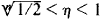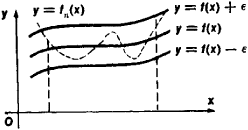# Uniform Convergence

Also found in: Wikipedia.

## uniform convergence

[′yü·nə‚fȯrm kən′vər·jəns]
(mathematics)
A sequence of functions {ƒn (x)} converges uniformly on E to ƒ(x) if given ε > 0 there is an N such that |ƒn (x) - ƒ(x)| < ε="" for="" all="">x in E provided n > N.
McGraw-Hill Dictionary of Scientific & Technical Terms, 6E, Copyright © 2003 by The McGraw-Hill Companies, Inc.
The following article is from The Great Soviet Encyclopedia (1979). It might be outdated or ideologically biased.

## Uniform Convergence

an important special case of convergence. A sequence of functions fn (x) (n = 1, 2, 3,…) is said to converge uniformly on a given set to the limit function f(x) if, for every ∊ > 0, there exists a number N = N(∊) such that, when η > N, ǀ f(x) – fn (x)ǀ < ∊ for all points x in the set.

For example, the sequence of functions fn(x) = xn converges uniformly on the closed interval [0, 1 /2] to the limit f(x) = 0. To show that this is true, let us take n > In (1/∊)/In 2. It then follows that ǀf(x)–fn (x)ǀ ≤ (1/2)n < ∊ for all x, 0 ≤ x ≤ 1/2. This sequence of functions, however, does not converge uniformly on the interval [0, 1]. Here, the limit function is f(x) = 0 for 0 ≤ x< 1 and f(1) = 1. The reason for the failure to converge uniformly is that for arbitarily large η there exist points η that satisfy the inequalitiesand for which ǀf (η) - fn(η)ǀ = ηn > 1/2.

The notion of uniform convergence admits of a simple geometric interpretation. The uniform convergence of a sequence of functions fn(x) on some closed interval to the function f(x) means that, for any ∊ > 0, all curves y = fn (x) with large enough n will be located within a strip that is 2∊ in width and is bounded by the curves y = f(x) ± ∊ for any x in the interval (see Figure 1).Figure 1

Uniformly converging sequences of functions have a number of important properties. For example, the limit of a uniformly converging sequence of continuous functions is also continuous. On the other hand, the example given above shows that the limit of a sequence of functions that does not converge uniformly may be discontinuous. An important role is played in mathematical analysis by Weierstrass’ theorem, which states that every function continuous on a closed interval can be represented as the limit of a uniformly converging sequence of polynomials. (SeeAPPROXIMATION AND INTERPOLATION OF FUNCTIONS.)

References in periodicals archive ?
Denote by H(D) the space of analytic functions on D endowed with the topology of uniform convergence on compacta.
As we mentioned in the introduction, Schwabik in  gives the next definition and proves some basic properties such as the Uniform Convergence Theorem.
Then we present the error analysis for the approximate solution and prove the uniform convergence in the discrete maximum norm.
* C([0, T], [R.sup.d]) the space of functions of [0, T] with values in Rd equipped with the topology of uniform convergence.
C([R.sub.+], [R.sub.+]) is the set of all continuous functions x : [R.sub.+] [right arrow] [R.sub.+], endowed with the topology of uniform convergence on compact subsets of [R.sub.+].
Furthermore, as known from Fourier Theory, [L.sub.2] convergence does not necessarily imply pointwise and uniform convergence. Here, however, in the PSWF reconstruction, [L.sub.2] convergence does imply uniform convergence and there is no Gibbs-like phenomenon.
When applied to the MPPT problem in PV systems, the method offers the benefit of uniform convergence behavior under a wide range of working conditions that includes temperature and irradiance variations and the non-symmetric power generation of the neighboring PV modules as a result of module degradation or mismatch.
In this section, we will prove the uniform convergence of the approximate solutions [S.sub.k](t) to the exact solution S(t).
Taking into account the expansion of the function g [??] [e.sup.[-1/2]g] entire series and using the uniform convergence of entire series we obtain the formula.
In this note we provide a new scheme via Chambolle's one  and show the locally uniform convergence of our scheme to the level set equation for (1) (cf.
The suitability of the chosen transformation method follows from the necessary condition for convergence, what can be deduced from theorem 2 and also from theorem 1, concerning the uniform convergence.

Site: Follow: Share:
Open / Close